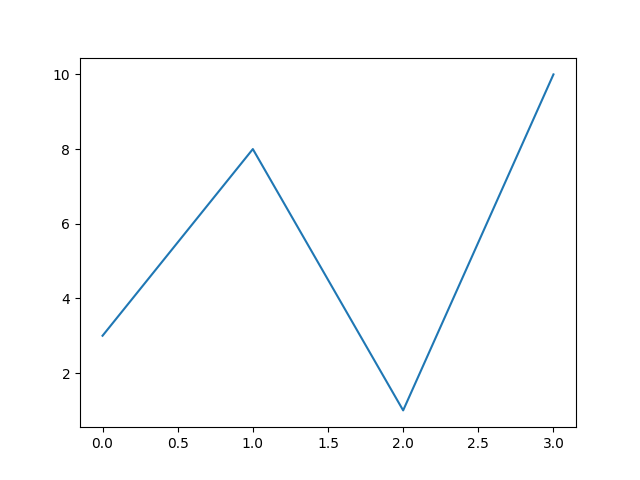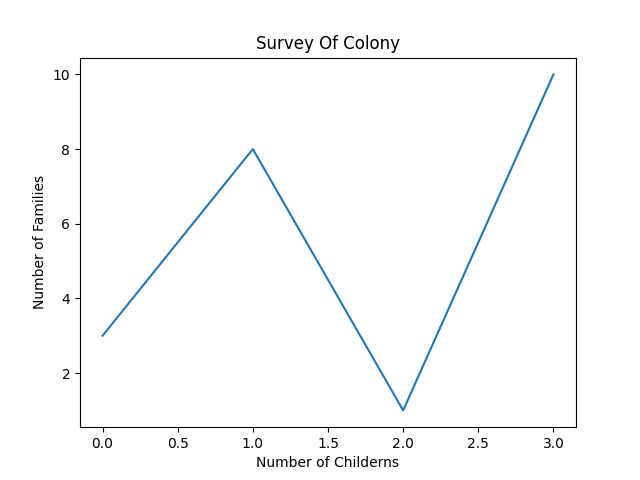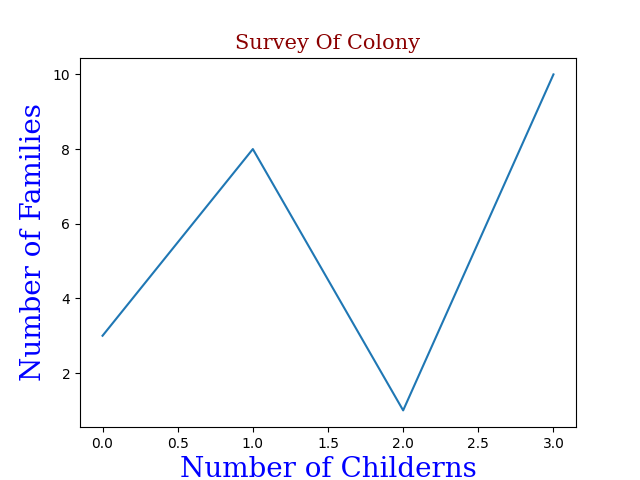# How to Add Labels in a Plot using Python?

• Difficulty Level : Medium
• Last Updated : 20 Oct, 2021

Prerequisites: Python Matplotlib

In this article, we will discuss adding labels to the plot using Matplotlib in Python. But first, understand what are labels in a plot. The heading or sub-heading written at the vertical axis (say Y-axis) and the horizontal axis(say X-axis) improves the quality of understanding of plotted stats.

Example: Let’s create a simple plot

## Python

 `# python program to plot graph without labels``import` `matplotlib``import` `matplotlib.pyplot as plt``import` `numpy as np`  `# it will take x coordinates by default``# starting from 0,1,2,3,4...``y ``=` `np.array([``3``, ``8``, ``1``, ``10``])` `plt.plot(y)``plt.show()`

Output:Plot without Labels or Title

## Creating Labels for a Plot

By using pyplot() function of library we can add xlabel() and ylabel() to set x and y labels.

Example: Let’s add Label in the above Plot

## Python

 `# python program for plots with label``import` `matplotlib``import` `matplotlib.pyplot as plt``import` `numpy as np`  `# Number of children it was default in earlier case``x ``=` `np.array([``0``, ``1``, ``2``, ``3``])` `# Number of families``y ``=` `np.array([``3``, ``8``, ``1``, ``10``])` `plt.plot(x, y)` `# Label for x-axis``plt.xlabel(``"Number of Childerns"``)` `# Label for y-axis``plt.ylabel(``"Number of Families"``)` `plt.show()  ``# for display`

Output:Plot with Labels

If you would like to make it more understandable, add a Title to the plot, by just adding a single line of code.

`plt.title("Survey Of Colony")`

Example:

## Python3

 `# python program for plots with label``import` `matplotlib``import` `matplotlib.pyplot as plt``import` `numpy as np`  `# Number of children it was default in earlier case``x ``=` `np.array([``0``, ``1``, ``2``, ``3``])` `# Number of families``y ``=` `np.array([``3``, ``8``, ``1``, ``10``])` `plt.plot(x, y)` `# Label for x-axis``plt.xlabel(``"Number of Childerns"``)` `# Label for y-axis``plt.ylabel(``"Number of Families"``)` `# title of the plot``plt.title(``"Survey Of Colony"``)` `plt.show()  ``# for display`

Output:Plot with Title

## Set Font Properties for Titles and Labels

In order to make the Plot more attractive use the fontdict parameter in xlabel(), ylabel(), and title() to apply the font properties.

## Python

 `# Adding font properties to labels and titles``import` `matplotlib``import` `matplotlib.pyplot as plt``import` `numpy as np`  `# Number of Children``x ``=` `np.array([``0``, ``1``, ``2``, ``3``])` `# Number of Families``y ``=` `np.array([``3``, ``8``, ``1``, ``10``])` `# label including this form1 will have these properties``form1 ``=` `{``'family'``: ``'serif'``, ``'color'``: ``'blue'``, ``'size'``: ``20``}` `# label including this form2 will have these properties``form2 ``=` `{``'family'``: ``'serif'``, ``'color'``: ``'darkred'``, ``'size'``: ``15``}` `plt.plot(x, y)``plt.xlabel(``"Number of Childerns"``, fontdict``=``form1)``plt.ylabel(``"Number of Families"``, fontdict``=``form1)``plt.title(``"Survey Of Colony"``, fontdict``=``form2)``plt.show()`

Output:Plot with Font Properties

My Personal Notes arrow_drop_up